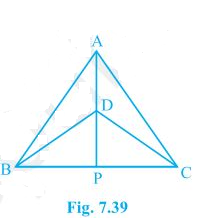# ΔABC and ΔDBC are two isosceles triangles on the same base BC and vertices A and D are on the same side of BC (see Fig.) . If AD is extended to intersect BC at P, show that (i) ΔABD ΔACD (ii) ΔABP ΔACP (iii) AP bisects A as well as D. (iv) AP is the perpendicular bisector of BC.

An Isosceles triangle is a triangle that has two equal sides. Also, the two angles opposite to the two equal sides are equal. In other words, we can say that “An isosceles triangle is a triangle which has two congruent sides”.#### Solution:

Given

Given: ∆ABC and ∆DCB are isosceles ∆on the same base BC.

To Prove

(i) ΔABD ≅ ΔACD

(ii) ΔABP ≅ ΔACP

(iii) AP bisects ∠A as well as ∠D.

(iv) AP is the perpendicular bisector of BC.

i) in ∆ABD and ∆ACB

AB=AC

BD=CD

∆ABD≅∆ACD ————-(By S.S.S congruency)

ii) in ∆ABP and ∆ACP

AB=AC

∠ BAP≅∠CAP [∆ABD≅∆ACD BY C.P.CT]

AP=AP ———[common]

∴[∆ABD≅∆ACD ———–[By S.A.S congruency ]

iii) [∆ABD≅∆ACD]

AP, bisects ∠A

In ∆ BDP and ∆DPB

BD=CD —————(GIVEN)

DP=PC ———-[∆AB≅ ∆ACP C.P.C.T]

DP=DP ———–[common]

∴∆BDP≅∆CDP (S.S.S)

∠BDP=∠CDP (C.P.C.T)

DP bisects ∠D

AP bisects ∠D ——(ii)

From above equations, AP bisects ∠ A as well as ∠ D

iv) ∠ AP +∠APC =180°

∠APB=∠APC ————-[∆ABP≅∆ACP C.P.CT]

∠APB + ∠APC=180°

2 ∠ APB=180°

∠APB=180/2=90°

BP=PC

∴AP is ⊥ bisects of BC.Articles to explore(3)(0)